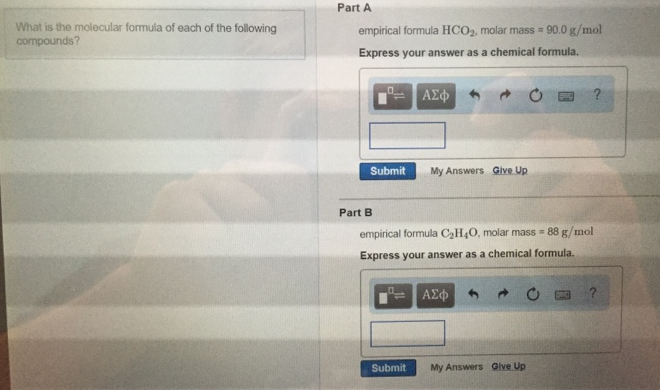# Problem: What is the molecular formula of each of the following compounds? Part Aempirical formula HCO2, molar mass = 90.0 g/mol. Express your answer as a chemical formula.Part Bempirical formula C2H4O, molar mass = 88 g/mol. Express your answer as a chemical formula.

###### FREE Expert Solution
91% (333 ratings)###### Problem Details

What is the molecular formula of each of the following compounds?

Part A

empirical formula HCO2, molar mass = 90.0 g/mol.

Part B

empirical formula C2H4O, molar mass = 88 g/mol.